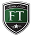The following video is borrowed from our BusinessTraining.com platform and was originally recorded for our financial modeling training program.  In the following video, you will receive a free lesson on financial and business math.

Video Transcript/SummaryThe strategies and tips provided within this video module include:

1. One of the most important calculations in finance is Net Present Value, which is the present value of future cash flows and works of the premise that cash in hand today is worth more than cash promised in the future. For example, if we assume future cash holding of \$1,000, inflation of 2.5% and a time horizon of 30 years, the present value is 1,000/(1+.0025)^30 = \$781
2. The discount rate (R) is essentially a measure of risk and can be adjusted to reflect the various types of risk such as default, credit risk, duration etc. This time periods for the rate of return needs to be matched with N.
3. Need to be aware of the difference between monthly and annual compounding and makes a material difference when dealing with larger sums. Consider \$1,000 20years from now at 12% interest = \$104. However, if we compound monthly for the same 20 year period, the NPV is reduced to \$91.8. Such an example highlights why credit card debt can be accumulated so quickly.
4. Discount factor is the factor by which you discount a certain cash flow (1/(1+r)^10. Future Value of money refers to the future value of money that you have today. Therefore the future amount of 1,000 today at 10% rate over 10 years is \$1,000 * (1+0.1)^10 = \$2,593.
5. In financial projections, there are multiple cash flow streams and an PV of each needs to calculated and added together in order to determine the NPV. This is the basis for a discounted cash flow model (DCF)
6. Many DCFs will use the end of year cash figure. However, cash will realistically flow into the business throughout the year so it may be more appropriate to use the mid-period assumption, resulting in discount factors of 0.5, 1.5, 2.5 etc.
7. The Internal Rate of Return (IRR) is another important figure which helps us determine which investment is most attractive and is essentially the discount rate that is used for a project. Sometime though, a lower IRR may make sense from a strategic/business perspective so we must use IRR as an aid rather than the final decision maker.

I hope that this video was a helpful overview of financial and business math.Your friends here at https://investmentcertifications.com